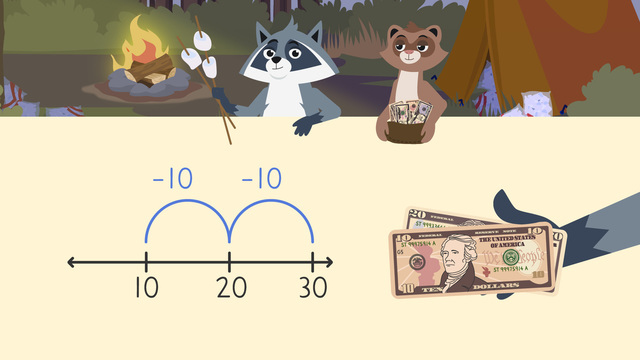# Making ChangeRating

Ø 4.5 / 4 ratings
The authorsTeam Digital

## Making Change

Sometimes when people are paying for items they do not have the exact amounts of money. When this happens, they pay more than they need to and receive change in return.

Money and making change is how many dollar bills or coins you get back when you pay too much. Change can be dollar bills or coins! It’s the difference of the money you pay with and how much the item costs.

## How to Make Change

There are different ways that you can figure out how to make change with money. In this video we used a number line while counting money and making change. We can learn about making change by counting up! When using a number line to subtract money, you can follow these steps:

Step # What to do
1 Start by creating an equation. You can subtract the amount of money
the item cost from how much money was given to pay for it.
2 Next, put the larger number on the right side of the number line.
3 Skip count backwards.
4 Finally, the number you land on is the answer, or the change!

## Making Change with Money – Example

Let’s practice making change with the example below.

Let's imagine we are buying a lot of supplies. If we pay one hundred dollars, and the supplies cost eighty-three dollars, how much change will we receive?

Let's start by setting up the equation! We know that we pay using $100 and we also know that the supplies cost$83. So in order to find out the change that remains, we set up a subtraction – 100 - 83. Now, let's solve our subtraction equation by taking steps back on the number line.If we subtract 80 from 100, we can take steps of 20 on our number line. We need to take 4 of these steps with steps of 20. So, subtracting 80 from 100, we land on 20 What remains is 3. So we need to subtract 3 from 20 to get to our result.

We will receive 17 dollars back in change!

## Making Change – More Practice

Remember, making change is the difference of how much you pay and how much the item costs. You can use many different tools to solve making change, such as a number line!

When using a number line to make change remember these steps:

Step # What to do
1 Start by creating an equation. You can subtract the amount of money the item cost from how much money was given to pay for it.
2 Next, put the larger number on the right side of the number line.
3 Skip count backwards.
4 Finally, the number you land on is the answer, or the change!

At the end of this making change video, there are interactive exercises for continued practice of making change through counting money and making change worksheets.

## Making Change exercise

Would you like to apply the knowledge you’ve learned? You can review and practice it with the tasks for the video Making Change .
• ### What are the steps for making change using a number line?

Hints

What do you need to create first?

Does the larger number go on the right of the number line before or after you count backward the amount of the second number?

Solution
• Create an equation.
• Put the larger number on the right.
• Count backward the amount of the second number.
• The final number is the change you receive back.
• ### Zuri and Freddie buy an umbrella.

Hints

Start on the right of the number line and skip count backward, or subtract the numbers on top of the number line.

What is 80 minus 6 groups of tens or 80 - 60?

What is 20 - 8?

Solution

• 80 - 60 = 20
• 20 - 8 = 12

• ### Time to buy boogie boards.

Hints

Start on the right of the number line and skip count backward, or subtract the numbers on top of the number line.

What is 150 minus 2 groups of fifty or 150 - 100?

What is 50 - 10?

What is 40 - 6?

Solution

• 150 - 100 = 50
• 50 - 10 = 40
• 40 - 6 = 34

• ### We need a camera!

Hints

Start on the right of the number line and skip count backward, or subtract the numbers on top of the number line.

What is 250 - 100?

What is 150 - 100?

What is 50 - 10?

Solution

• 250 - 100 = 150
• 150 - 100 = 50
• 50 - 10 = 40
• 40 - 8 = 32
They will receive \$32 in change.